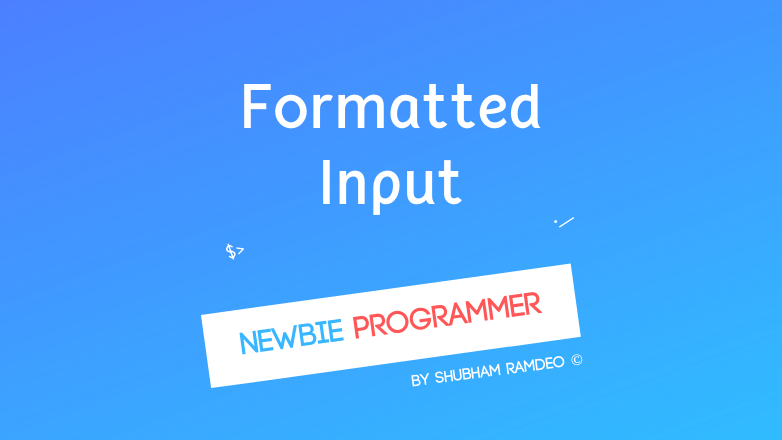# Basic Formatted Input

PUBLISHED Jul 14th, 2015

That’s how computer hears, using keyboard to take input, formatted input !Welcome to the Newbie Programmer Series. In the last part, we have discussed how to print formatted messages on the screen etc. In this post we will discuss how to take formatted input from the user through the keyboard. So if you are new to this series, please go to the index (here) and read out all the previous parts so that you can easily understand what’s going down below.

### Formatted Input using scanf

For taking anything from the keyboard, we can use scanf :

scanf (“ input format “, variables );

Here, for now, we will use a with the name of the variables.

For example :

``````#include <stdio.h>
main()
{
int num;
scanf("%d", &num);
printf("You entered : %d \n", num);
}
``````

The above code works as follows :

• A variable num is created in the computer’s memory.
• Using scanf, the user is required to enter something from keyboard. Here we have used %d which means a user have to enter some number. And after he presses ENTER key, the number he has entered is saved in the variable ‘num’.
• With printf, “You entered : %d” is shown on the screen, where %d is replaced by the number, in the variable num, which the user has entered.

So the Output is :

``````32
You entered : 32
``````

For taking a fractional value, you can use %f, for a character, use %c.

You can make cute calculating programs like this :

``````#include <stdio.h>
main()
{
int a, b;
printf("Enter two numbers one by one \n");
scanf("%d", &a);
scanf("%d", &b);
printf("The sum is %d \n", a + b );
}
``````

Output :

``````Enter two numbers one by one
10
20
The sum is 30
``````

But as the heading says “formatted input”, what you can take input just as printf outputs. Just remember, %d etc are to be replaced by the Input or Output. Like this one :

``````#include <stdio.h>
main()
{
int a, b;
printf("Enter two Numbers \n");
scanf("%d %d", &a, &b);
printf("The sum is %d", a + b);
}
``````

Compare the above two codes, in this one, the user will enter two numbers with together and a space between them, separating each. And each of the %d is replaced by the value entered by the user and will be saved in the a and b variables. So output is like :

``````Enter two Numbers
12 10
The sum is 22
``````

You can enter inputs in date or time formats too :

``````#include <stdio.h>
{
int hr, min;
printf("Enter Time : ");
scanf("%d:%d", hr, min);
printf("Hour is %d, Minute is %d", hr, min);
}
``````

Look, I have not used \n in the “Enter Time : “,  So now I can enter in the same line as :

``````Enter Time : 2:30
Hour is 2, minute is 30
``````

The thing is simple. We first make some variable. Take some input using scanf, calculate something and use printf to print the result.

This remind me of a calculator that I made while learning programming :

``````// Simple Calculation
#include <stdio.h>
main()
{
float a, b;
printf("Enter two numbers with a space between them \n");
printf("*Please don't enter zero as second number ! \n");
scanf("%f %f", &a, &b);
printf("Sum is %f \n", a + b);
printf("Difference is %f \n", a - b);
printf("Product is %f \n", a * b);
printf("Division is %f \n", a /b );
}
``````

See I have used %f so that the user can enter any number, including fractions. As division by zero is not possible, user should not enter zero otherwise this program will stop ! So the Output is like :

``````Enter two numbers with a space between them
*Please don't enter zero as second number !
200 50
sum is 250.0000
Difference is 150.0000
Product is 10000.0000
Division is 4.0000
``````

Cute project huh ! <3

I think it’s enough for today. In the next post, we will do some more interesting stuffs. If any doubt, please comment below. Thanks for reading, Stay Connected ! :)

#### You might also like:

(prev)
(next)

(rand) Variables a handbag to store data!
(rand) Wordpress to Jekyll - Jekyll Exporter plugin
(rand) Open Source in a minute